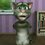# Is the trigonometry we are doing correct?

I am considering $\cos\theta=\sec\theta$then $\cos\theta=\frac{1}{\cos\theta}$ $=>\cos^2\theta=1$ $=>\cos\theta=1$ $=>\theta=0^\circ$here $\sin\theta=\sin 0^\circ=0.$ the next way again consider $\cos\theta=\sec\theta$ $=>\frac{adj}{hyp}=\frac{hyp}{adj}$ $=>\frac{adj^2}{hyp^2}=1$ $=>\cos^2\theta=1$ $=>\cos\theta=-1$ nothing too interesting hereNote by Sudoku Subbu
6 years, 6 months ago

This discussion board is a place to discuss our Daily Challenges and the math and science related to those challenges. Explanations are more than just a solution — they should explain the steps and thinking strategies that you used to obtain the solution. Comments should further the discussion of math and science.

When posting on Brilliant:

• Use the emojis to react to an explanation, whether you're congratulating a job well done , or just really confused .
• Ask specific questions about the challenge or the steps in somebody's explanation. Well-posed questions can add a lot to the discussion, but posting "I don't understand!" doesn't help anyone.
• Try to contribute something new to the discussion, whether it is an extension, generalization or other idea related to the challenge.

MarkdownAppears as
*italics* or _italics_ italics
**bold** or __bold__ bold
- bulleted- list
• bulleted
• list
1. numbered2. list
1. numbered
2. list
Note: you must add a full line of space before and after lists for them to show up correctly
paragraph 1paragraph 2

paragraph 1

paragraph 2

[example link](https://brilliant.org)example link
> This is a quote
This is a quote
    # I indented these lines
# 4 spaces, and now they show
# up as a code block.

print "hello world"
# I indented these lines
# 4 spaces, and now they show
# up as a code block.

print "hello world"
MathAppears as
Remember to wrap math in $$ ... $$ or $ ... $ to ensure proper formatting.
2 \times 3 $2 \times 3$
2^{34} $2^{34}$
a_{i-1} $a_{i-1}$
\frac{2}{3} $\frac{2}{3}$
\sqrt{2} $\sqrt{2}$
\sum_{i=1}^3 $\sum_{i=1}^3$
\sin \theta $\sin \theta$
\boxed{123} $\boxed{123}$

Sort by:

Note: $\cos ^2 \theta = 1 \not \Rightarrow \cos \theta = 1$. Do you see why?

Staff - 6 years, 6 months ago

why staff iam sending the sqare to that side so $\cos\theta=1$ hmmm.. ttooo simple concept

- 6 years, 6 months ago

If $x^2 = 1$, is $x = 1$ the only solution?

Staff - 6 years, 6 months ago

ya ya i understand your concept if $x^2=1$ then x may be -1 or +1 thanks calvin i'll change

- 6 years, 6 months ago

Right, that is a very common mistake made when trying to solve such equations.

The best approach would be to say $0 = x^2 - 1 = (x-1)(x+1)$ and hence $x = 1, -1$.

Staff - 6 years, 6 months ago

thanks for your help visit my other notes and reply if there is any mistake

- 6 years, 6 months ago

We can't say "concept" when we talk about "Fundamental theorem of algebra".

- 6 years, 5 months ago

Another thing... you don't just consider the first quadrant angle.. Consider also its coterminal angles..

- 6 years, 6 months ago

Remember the Pythagorean Identity: $\sin^2{\theta}+\cos^2{\theta}=1$ If $\cos^2{\theta}=1$, this implies that: $\sin^2{\theta}=0 \\ \sin{\theta}=0$ ¿For what angles is this true? For any angle of the form $\theta=n\pi$ As can be easily verified seeing any graph of the sine function.

- 6 years, 6 months ago

boss what r u trying to tel??

- 5 years, 9 months ago